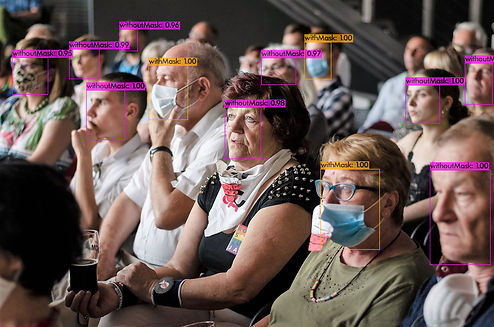top of pageOn this website you can try the model with your own images and buy it if you are satisfied with its accuracy.

Model description:

This model detects  the wearing / non-wearing or improper wearing of a medical mask.

Metrics:

detections_count = 883, unique_truth_count = 496
class_id = 0, name = withoutMask, ap = 81.00%            (TP = 60, FP = 16)
class_id = 1, name = withMask, ap = 92.71%       (TP = 373, FP = 31)
class_id = 2, name = maskWearedIncorrect, ap = 77.35%            (TP = 13, FP = 8)

for conf_thresh = 0.25, precision = 0.89, recall = 0.90, F1-score = 0.89
for conf_thresh = 0.25, TP = 446, FP = 55, FN = 50, average IoU = 73.17 %

IoU threshold = 50 %, used Area-Under-Curve for each unique Recall
mean average precision (mAP@0.50) = 0.836853, or 83.69 %

System requirements:

Inference time using CPU: 300 ms (on HP Laptop 15-DA0042NH (Processor: Intel(R) Core(TM) i7-8550U CPU))

bottom of page